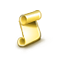Schau, wie man Roboter kostenlos herunterladen kann

Interessantes Skript?
Veröffentliche einen Link auf das Skript, damit die anderen ihn auch nutzen können

Hat Ihnen das Skript gefallen?
Bewerten Sie es im Terminal MetaTrader 5# Bracket Order with Correlation Adjusted Position Size - Skript für den MetaTrader 4

Ansichten:
34
Rating:
Veröffentlicht:
2020.02.11 12:45
Aktualisiert:
2020.02.12 17:21

These scripts calculate the correlation coefficient to all other open positions in other symbols and adjusts the position size by a well tested formula.

In addition to that, It will also consider if you have full position size or not. In order to memorize the initial stoploss, it will save the initial stop loss in the order comment. If there's orders opened without the script it will take the average of all stoplosses and entryprices, weighted to lotsize.

It is very useful if you have a system with standardized stops, based on volatility (ATR, Standard Deviation, Basis Points, or even Pips for similar underlyings such as EURUSD and GBPUSD).

The advantage is simply, that you will never oversize again due to a correlation added risk

Settings:

• Stop Multiplier (1.0 = unchanged) [1.0]: If you want to have a stop twice the size as the 1x Standard Deviation, with settings below, then you use 2.0. If you want half the standard deviation 0.5 etc.
• Lotsize Multiplier [1.0]: If you want to risk more than the stated Risk as Riskpercentage or Riskamount (see below), then change this to a multiple that you like. 2.0 for twice the risk, e.g. 5% --> 10%
• Risk to Reward Multiplier (1x Target) [2.0]: This will set the target as a multiple of your stoploss distance. The standard setting is a 1:2 Risk-to-Reward target
• Standard Deviation Period for Stoploss : The Period for the Smoothed 1x Standard Deviation, which is the base for the stoploss distance calculation. 30 Periods f.e. mean that it will take the standard deviation of close prices of the last 30 candles on the chart
• Risk in Percentage ON/OFF [ON]: If this is activated with "ON", then it will take the Riskpercentage of Accountbalance, specified below, as the money at risk for your trade, when it is fully stopped out. It will always take the exact risk or less, never more than specified.
• Riskpercentage of AccountBalance [5.0]: Risk in Percentage of Accountbalance for each Trade. 5.0 means 5% of \$1,000 f.e. is \$50 risk per trade.
• Riskamount if Riskpercentage OFF [50.0]: If Riskpercentage is turned "OFF", then this is the exact amount of risk in your account currency. 50.0 is \$50 risk f.e.
• Magic Number : The script will use the magic number to target previously opened trades by the script (one of the scripts, so numbers should be same) to know the initial stoploss distance for correlation "relevance" calculation.Dax_EA

Läuft seit 1.1.2019 auf dem Echtgeldkonto. Ja, das Konto ist noch nicht im Minus und wird es auch nicht werden :-) EA läut stabil und nur im Dax.Slow_But_Steady

EA needs with no indicator, but smart strategyJMA_CCI

Ein weiterer Indikator Jurika.Ind-SKB-1

Indikator Ind-SKB-1.